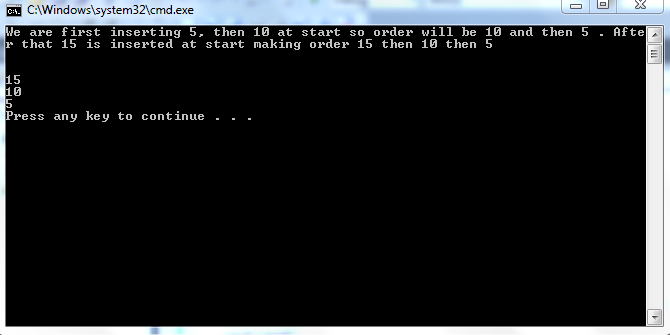# Data Structure using C++

In this tutorial, you will learn implementation of following functions 1. Constructor 2. Checkempty() 3. Traversal 4. Insertion at beginning Along with this tutorial, C++ code is given which has structure node and class Linklist containing these codes with a main to help you in understanding how these functions are being used. What is the code of constructor? The code of the constructor utilizes the fact that we want to make a check empty function which returns 1 if linked list has no element and 0 if it has elements. So we simply make the head point to NULL. It means that if a new linked list is made, its head points to NULL and this can be used for checkempty()
` Linklist(){ head=NULL;} `
Given above is the code of linked list. What is the code of checkempty() function? The code of checkempty() function uses the fact that head is initially initialized with NULL.
`Bool checkempty(){ if (head==NULL)       return 1;else        return 0;    }`
Given above is the code for checkempty(). In this function we are checking whether the head is null or not. If it is we simply return1 otherwise we return 0. What is the code of Traverse function?
`void Traverse(){ if (checkempty()){cout<<"List is empty";}else{Node * curr;curr=head;while(curr!=NULL){ 		cout<<curr->data; 		cout<<endl;curr=curr->next;}} } `
First of all we check if list is empty by calling the checkempty function, if it is, we stop the traversal and if it is not, we make a curr pointer pointing to first element and move it at the end until last element is not reached. What is the code for inserting in the beginning of a linked list?
`void InsertAtStart(int val){Node *nnode=new Node;nnode->data=val;nnode->next=head;head=nnode;}`
Here a pointer pointing to a dynamic node is made. First of all value of data is given and then as it is inserted in start, its next must be previous first. So its next is made equal to head of the linked list. For already empty linked list, head is NULL so its next will point to NULL which means it is the only (first and last) node. Hence, logic is true for all cases. Note, we are making dynamic nodes so destructor is necessary. In the next tutorial, implementation of some other basic functions will be discussed. And after that, we will use of these functions to make functions we want like insertion at a specified location. Output: